# Online GCSE Math

## Get 1 on 1 help from GCSE Math tutors

General Certificate of Secondary Education (GCSE) math course is specifically designed for learners who want to pass GCSE syllabus exams. The GCSE math syllabus covers simple to complex topics. A student studying for the GCSE exam would begin by learning basic mathematical skills then proceed to more advanced topics. The design of this course is to help the learner acquire mathematical knowledge through a progressive ladder. It makes learning mathematical skills easy because the student begins from simple concepts to complex concepts.

### GCSE Math

General Certificate of Secondary Education (GCSE) math course is specifically designed for learners who want to pass GCSE syllabus exams. The GCSE math syllabus covers simple to complex topics. A student studying for the GCSE exam would begin by learning basic mathematical skills then proceed to more advanced topics. The design of this course is to help the learner acquire mathematical knowledge through a progressive ladder. It makes learning mathematical skills easy because the student begins from simple concepts to complex concepts. Nearly everyone requires mathematical skills covered in the GCSE syllabus in daily life. The basic mathematical concepts often apply in our daily lives.

### What to expect in GCSE Math Problem – Topics of GCSE

#### Inequalities

Under this unit, the learner would learn fundamental subtopic in algebra and learn  how to solve apply the solution of quadratic equation, simultaneous equation of one or two variables.

#### Sequence

Under this unit, the learner would learn rules such as term-to-term rules. Notable operations are quadratic sequences and linear sequences, and geometric progression, and Fibonacci sequences.

#### Ratio, proportion and rate of change

In this unit, the student will cover topics such as scale factors, ratio notation, diagrams, and maps. The student will be taught skills or methods  of reducing ratios into the simplest forms.

#### Graphs

Under this unit, the learner would cover topic such as basic graphs coordinates form algebraic expression for a graph,  plot graphs such as parallel lines graph, eclipse, and hyperbola.

#### Geometry and Measures

In this unit, the learner would cover topics such as vertices, lines, planes, perpendicular lines, parallel lines, triangles, and polygons. By the end of this unit, the learner should be able to demonstrate geometry theorems.

#### Vectors

This topic equips the learner will knowledge and skills in solving problems involving vector equations. The learner would be taught to solve two or three vectors.

#### Probability and Statistics

The units expose the learner to learn the probability equation. The learner would learn concepts such as the frequency of a given distribution and outcome of events. After covering this unit, the learner should be able to apply ideas such as randomness, fairness, and likely events to solve mathematical problems. Further, the unit also covers distribution, sampling, mean, median and mode.

It is important to know what to expect as you prepare for GCSE math because it would affect the way you will perform in this subject. A good student should at least have a plan on how to tackle the problems that he or she would face in GCSE math. Here are a few tips on how to prepare and revise for the GCSE math. You should revise concepts and units learned continuously because it helps you to rivet and master skills that you have learned in class.

It is important to try to memorize the concept that you learn. You know which resources are important for the revision exercise because the quality of the materials used affect the learning process and mastery of the subject. You should breakdown the problems into small units so that you may develop skills over time. You should be able to ask yourself how difficult the problem is so that you go deeper with your revision.

There are so many resources available online for GCSE, which can help you as you study this course. The online resources for GCSE math include online expert tutors, revision materials, and books. You need to concentrate on tackling concepts that you have not comprehended during class activities.

Importantly, remember to seek help with your math revision from the onset of the course. Do not wait until the last minute to begin your revision because the content of this course is complex hence you need to progressively learn the topics as you cover them in class.Same Tutor
Every TimeTutors with
MS DegreeSame
TextbooksManaged
TutoringVery
ConvenientAffordable
\$19/hr for 8hr Monthly
Below 8hr/Month, \$20/hr
What You’ll Learn
• Inequalities
• Sequence
• Graphs
• Ratio, proportion and rate of change
• Geometry and Measures
• Vectors
• Probability and Statistics
• Fractions, percentages and decimals
• Measures and accuracy
• Algebra•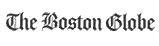•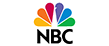••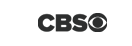•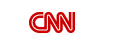•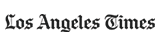•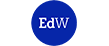•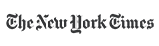•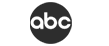### How You Benefit FromThis Program

• Flexible Scheduling
• Quality Learning
• Proven Results
• Tutors with Masters
• Affordable Price
• Dedicated Support
• Money Back Guarantee

Name*
Phone number*
Email*